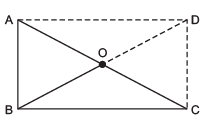Courses

# NCERT Solutions(Part- 4)- Understanding Quadrilaterals Class 8 Notes | EduRev

## Class 8 : NCERT Solutions(Part- 4)- Understanding Quadrilaterals Class 8 Notes | EduRev

The document NCERT Solutions(Part- 4)- Understanding Quadrilaterals Class 8 Notes | EduRev is a part of the Class 8 Course Class 8 Mathematics by VP Classes.
All you need of Class 8 at this link: Class 8

EXERCISE  3.4

Question 1. State whether True or False.
(a) All rectangles are squares.
(b) All rhombuses are parallelograms.
(c) All square are rhombuses and also rectangles.
(d) All squares are not parallelograms.
(e) All kites are rhombuses.
(f) All rhombuses are kites.
(g) All parallelograms are trapeziums.
(h) All squares are trapeziums.
Solution: (a) False

(b) True
(c) True
(d) False
(e) False
(f) True
(g) True
(h) True

Question 2. Identify all the quadrilaterals that have: (a) Four sides of equal length. (b) Four right angles.
Solution: (a) Squares as well as rhombus have four sides of equal length. (b) Squares as well as rectangles have four right-angles.

Question 3. Explain how a square is:
(ii) a parallelogram
(iii) a rhombus
(iv) a rectangle

Solution:
(i) A square is a 4 sided figure, so it is a quadrilateral.
(ii) The opposite sides of a square are equal and parallel, so it is a parallelogram.
(iii) All the sides of a square are equal, so it is a rhombus.
(iv) Each angle of a square is a right angle, so it is a rectangle.

Question 4. Name the quadrilaterals whose diagonal:
(i) bisect each other
(ii) are perpendicular bisectors of each other
(iii) are equal
Solution: (i) The diagonals of the following quadrilaterals bisect each other:
Parallelogram, rectangle, square, rhombus
(ii) The diagonals are perpendicular bisectors of the following quadrilaterals:
Square and rhombus
(iii) The diagonals are equal in case of:
Square and rectangle

Question 5. Explain why a rectangle is a convex quadrilateral.
Solution: (i) All the angles have measures less than 180°.

(ii) Both diagonals lie wholly in the interior of the rectangle.
∴ The rectangle is a convex quadrilateral.

Question 6. ABC is a right-angled triangle and O is the mid-point of the side opposite to the right angle. Explain why O is equidistant from A, B and C. (The dotted lines are drawn additionally by to help you.)Solution: Produce BO to D such that BO = OD.
Joining CD and AD, we get a quadrilateral ABCD in which opposite sides are parallel.
∴ ABCD is a parallelogram.
∠ABC = 90°
∴  ABCD is a rectangle.
Since, diagonals of a rectangle bisect each other, i.e. O is the mid-point of BD and AC ∴ O is equidistant from A, B and C.

Question 7. A mason has made a concrete slab. He needs it to be rectangular. In what different ways can he make sure that it is rectangular?
Solution: He can make sure that it is a rectangular using the following different ways:
(i) By making opposite sides of equal length.
(ii) By keeping each angle at the corners as 90°.
(iii) By keeping the diagonals of equal length.
(vi) By making opposite sides parallel and ensuring one angle as 90° in measure.
(v) By making all angles equal and ensuring the measure of one angle as 90°.

Question 8. A square was defined as a rectangle with all sides equal. Can we define it as rhombus with equal angles? Explore this idea.
Solution: Yes, because a rhombus becomes a square if its all angles are equal.

Question 9. Can a trapezium have all angles equal? Can it have all sides equal? Explain.
Solution: Yes, if it is a rectangle. It can have all sides equal, when it becomes a square.

Offer running on EduRev: Apply code STAYHOME200 to get INR 200 off on our premium plan EduRev Infinity!

93 docs|16 tests

,

,

,

,

,

,

,

,

,

,

,

,

,

,

,

,

,

,

,

,

,

;